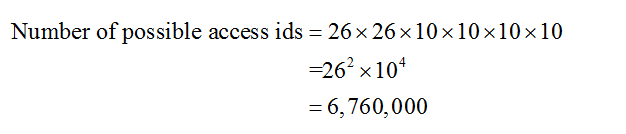# Every Wayne State student has an access id; the 6-character name consisting of 2 letters (a-z) followed by 4 numbers (0-9).a. How many access ids are possible?b. How many access ids are there where letters and numbers are not repeated?c. How many access ids are there where letters and numbers are not repeated and the id only contains odd numbers?

Question
57 views

Every Wayne State student has an access id; the 6-character name consisting of 2 letters (a-z) followed by 4 numbers (0-9).

a. How many access ids are possible?

b. How many access ids are there where letters and numbers are not repeated?

c. How many access ids are there where letters and numbers are not repeated and the id only contains odd numbers?

check_circle

Step 1

a) Calculating the total number of ways:

The access id consist 6 character name consisting 2 letters followed by 4 numbers.

Each of the two letters has 26 possibilities. Four numbers (not necessarily distinct) are selected from the 10 numbers. Since the repetition is allowed, each of the numbers 5 numbers has 10 possibilities. The required number of ways is calculated as follows:Step 2

b) Calculating the total number of ways:

Here, numbers and letters are not repeated. First and second letters have 26 and 25 possibilities respectively. Four distinct numbers are selected from the 10 numbers. That is, first number has 10 possibilities, second number has 9, third number has 8 and fourth number has 7 poss...

### Want to see the full answer?

See Solution

#### Want to see this answer and more?

Solutions are written by subject experts who are available 24/7. Questions are typically answered within 1 hour.*

See Solution
*Response times may vary by subject and question.
Tagged in

### Statistics